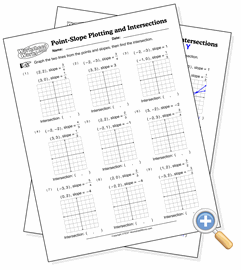# Point-Slope Plotting and IntersectionsGraph lines with a point and slope, then find the intersecting point

This is a multi-step exercise involving points and slopes. Students must draw two lines on the graph using points and slopes, and then find the point where they intersect. If they plot the lines correctly, the intersecting point will exist on the grid and have integer values.